﻿ 基于博弈策略下的新冠模型研究：以印度为例

# 基于博弈策略下的新冠模型研究：以印度为例A COVID-19 Model Based on Game Theory: A Case of India

2019年底，新型冠状病毒肺炎出现并快速成为全球流行疾病，不但对我们的身体健康造成威胁，对社会经济发展也带来了巨大影响。作为最有效的控制策略，疫苗接种可以通过减少易感者来控制疾病的传播。而接种疫苗会产生一定的经济支付和疫苗副作用风险，不接种疫苗则需承担染病风险以及感染的治疗费用。所以引入博弈免疫策略，可以使得人们通过衡量自身收益来决定是否接种疫苗，从而结合经济情况做出有利于自身的决策。本文建立了SEIQR的传染病动力学模型，引入博弈免疫策略，计算其基本再生数、有效再生数和平衡点，分析其动力学行为。运用印度2020年4月1日至2020年7月29日期间的新增病例和累计病例数据，结合模型进行数值模拟，结果表明在按比例接种疫苗的情况下，当出生率与接种率比例达到一定值时，就能够有效控制疾病传播，并且接种比例越大，疾病流行时间会越短，染病人数也会越少。同时在自愿接种疫苗政策下博弈免疫策略也是有效的，当人们学习模仿能力强或者不接种疫苗个体感染疾病的可能性更大时，会促使更多人接种疫苗，疾病会更快得以控制，并且能够保证人们自身利益。

Abstract: At the end of 2019, COVID-19 emerged and quickly became a global pandemic, posing a threat not only to our physical health but also to social and economic development. As the most effective control strategy, vaccination can control the spread of the disease by reducing the number of vulnerable people. Vaccination will produce certain economic costs and risks of side effects, while non-vaccination will bear the risk of infection and the cost of treatment of infection. Therefore, the introduction of game immunity strategy can enable people to decide whether to vaccinate or not by measuring their own benefits, so as to make favorable decisions based on the economic situation. In this paper, a SEIQR model was established, and game immune strategy was introduced to calculate the basic reproductive number, effective reproductive number and equilibrium point, and analyze its dynamic behavior. Using India on April 1, 2020 to July 29, 2020 new cases and cases accumulated data, numerical simulation combined with model, the results show that in the case of proportional vaccination, when the ratio of birth rate to vaccination rate reaches a certain value, the spread of the disease can be effectively controlled, and the larger the vaccination rate, the shorter the epidemic time of the disease and the fewer the number of infected patients. At the same time, it is concluded that the game immunization strategy is also effective under the voluntary vaccination policy. When people have a strong learning ability to imitate or individuals who are not vaccinated are more likely to contract the disease, more people will be vaccinated and the disease will be controlled faster, and people’s own interests are also protected at this time.

1. 引言

2. 模型

2.1. 模型建立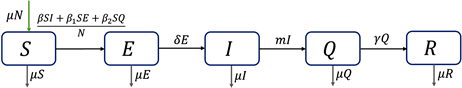Figure 1. The flow chart of COVID-19 propagation considering birth and death

$\left\{\begin{array}{l}\frac{\text{d}S}{\text{d}t}=\mu N-\frac{\beta SI+{\beta }_{1}SE+{\beta }_{2}SQ}{N}-\mu S,\\ \frac{\text{d}E}{\text{d}t}=\frac{\beta SI+{\beta }_{1}SE+{\beta }_{2}SQ}{N}-\delta E-\mu E,\\ \frac{\text{d}I}{\text{d}t}=\delta E-mI-\mu I,\\ \frac{\text{d}Q}{\text{d}t}=mI-\gamma Q-\mu Q,\\ \frac{\text{d}R}{\text{d}t}=\gamma Q-\mu R.\end{array}\text{}$ (1)

2.2. 引入博弈免疫策略

${f}_{v}=-{r}_{v}.$

${f}_{n}=-{r}_{i}\eta \left(E\left(t\right)+I\left(t\right)+Q\left(t\right)\right),$

$\Delta E={f}_{v}-{f}_{n}.$

$\frac{\text{d}x}{\text{d}t}=\left(1-x\right)\cdot \sigma x\cdot \rho \Delta E,$

$\Delta E<0$ 时，疫苗接种者可能会转换为非疫苗接种者，此时有

$\frac{\text{d}x}{\text{d}t}=-x\cdot \sigma \left(1-x\right)\cdot \rho \left(-\Delta E\right).$

$\frac{\text{d}x}{\text{d}t}=kx\left(1-x\right)\left({f}_{v}-{f}_{n}\right)=kx\left(1-x\right)\left[-{r}_{v}+{r}_{i}\eta \left(E+I+Q\right)\right].$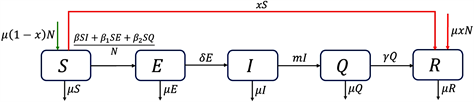Figure 2. The flow chart of COVID-19 propagation with game immune strategy

$\left\{\begin{array}{l}\frac{\text{d}S}{\text{d}t}=\mu \left(1-x\right)N-\frac{\beta SI+{\beta }_{1}SE+{\beta }_{2}SQ}{N}-xS-\mu S,\\ \frac{\text{d}E}{\text{d}t}=\frac{\beta SI+{\beta }_{1}SE+{\beta }_{2}SQ}{N}-\delta E-\mu E,\\ \frac{\text{d}I}{\text{d}t}=\delta E-mI-\mu I,\\ \frac{\text{d}Q}{\text{d}t}=mI-\gamma Q-\mu Q,\\ \frac{\text{d}R}{\text{d}t}=\mu xN+xS+\gamma Q-\mu R,\\ \frac{\text{d}x}{\text{d}t}=\kappa x\left(1-x\right)\left[-1+\omega \left(E+I+Q\right)\right].\end{array}\text{}$ (2)

3. 2.2中模型(2)的分析

3.1. 没有人接种疫苗，即x = 0

$F=\left[\begin{array}{ccc}\beta & {\beta }_{1}& {\beta }_{2}\\ 0& 0& 0\\ 0& 0& 0\end{array}\right],\text{}V=\left[\begin{array}{ccc}\delta +\mu & 0& 0\\ -\delta & m+\mu & 0\\ 0& -m& \gamma +\mu \end{array}\right],$

${V}^{-1}=\left[\begin{array}{ccc}\frac{\text{1}}{\delta +\mu }& 0& 0\\ \frac{\delta }{\left(\delta +\mu \right)\left(m+\mu \right)}& \frac{1}{m+\mu }& 0\\ \frac{\delta m}{\left(\delta +\mu \right)\left(m+\mu \right)\left(\gamma +\mu \right)}& \frac{m}{\left(m+\mu \right)\left(\gamma +\mu \right)}& \frac{1}{\gamma +\mu }\end{array}\right],$

${R}_{\text{0}}=\rho \left(F{V}^{-1}\right)=\frac{\delta \left(\gamma +\mu \right)\beta +\left(m+\mu \right)\left(\gamma +\mu \right){\beta }_{1}+\delta m{\beta }_{2}}{\left(\gamma +\mu \right)\left(m+\mu \right)\left(\delta +\mu \right)}.$

$s=\frac{S}{N},\text{}e=\frac{E}{N},\text{}i=\frac{I}{N},\text{}q=\frac{Q}{N},\text{}r=\frac{R}{N},$

$\left\{\begin{array}{l}\frac{\text{d}s}{\text{d}t}=\mu -\left(\beta si+{\beta }_{1}se+{\beta }_{2}sq\right)-\mu s,\\ \frac{\text{d}e}{\text{d}t}=\beta si+{\beta }_{1}se+{\beta }_{2}sq-\delta e-\mu e,\\ \frac{\text{d}i}{\text{d}t}=\delta e-mi-\mu i,\\ \frac{\text{d}q}{\text{d}t}=mi-\gamma q-\mu q.\end{array}\text{}$ (3)

${D}_{1}=\left\{\left(s,e,i,q\right)\in {R}^{4}|0\le s,e,i,q\le 1,0\le s+e+i+q\le 1\right\}$

${D}_{1}$ 为正向不变集。

${\zeta }_{2}\left({s}^{*},{e}^{*},{i}^{*},{q}^{*}\right)=\left(\frac{1}{{R}_{0}},\frac{\mu \left({R}_{0}-1\right)}{\left(\delta +\mu \right){R}_{0}},\frac{\delta \mu \left({R}_{0}-1\right)}{\left(\delta +\mu \right)\left(m+\mu \right){R}_{0}},\frac{\delta m\mu \left({R}_{0}-1\right)}{\left(\delta +\mu \right)\left(m+\mu \right)\left(\gamma +\mu \right){R}_{0}}\right)$.

2) 当 ${R}_{0}>1$ 时，地方病平衡点 ${\zeta }_{2}$ 在正向不变集 ${D}_{1}$ 内是全局渐近稳定的。

$M=F-V=\left(\begin{array}{ccc}{\beta }_{1}-\delta -\mu & \beta & {\beta }_{2}\\ \delta & -\mu & 0\\ 0& 0& -\gamma -\mu \end{array}\right),$

${R}_{0}>1⇔S\left(M\right)>0,\text{}{R}_{0}<1⇔S\left(M\right)<0.$

${\zeta }_{1}$ 的雅可比矩阵为

$J\left({\zeta }_{1}\right)=\left(\begin{array}{cc}M& 0\\ {J}_{1}& {J}_{2}\end{array}\right),$

${J}_{1}=-F,\text{\hspace{0.17em}}\text{\hspace{0.17em}}{J}_{\text{2}}\left({\zeta }_{1}\right)=\left(\begin{array}{cc}-\mu & 0\\ 0& -\mu \end{array}\right).$

${J}_{\text{2}}$ 的特征值为 ${\lambda }_{\text{1}}={\lambda }_{2}=-\mu$。当 ${R}_{0}<1$ 时， $S\left(M\right)<0$$S\left({J}_{\text{2}}\left({\zeta }_{1}\right)\right)<0$。所以无病平衡点 ${\zeta }_{1}$${R}_{0}<1$ 时局部渐近稳定。

${L}_{1}=s-{s}^{0}-{s}^{0}\mathrm{ln}\frac{{s}^{0}}{s}+e+\left[\frac{\beta {s}^{0}}{m+\mu }+\frac{m{\beta }_{2}{s}^{0}}{\left(m+\mu \right)\left(\gamma +\mu \right)}\right]i+\frac{{\beta }_{2}{s}^{0}}{\gamma +\mu }q,$

$\begin{array}{c}\frac{\text{d}{L}_{1}}{\text{d}t}=\left(1-\frac{{s}^{0}}{s}\right){s}^{\prime }+{e}^{\prime }+\left[\frac{\beta {s}^{0}}{m+\mu }+\frac{m{\beta }_{2}{s}^{0}}{\left(m+\mu \right)\left(\gamma +\mu \right)}\right]{i}^{\prime }+\frac{{\beta }_{2}{s}^{0}}{\gamma +\mu }{q}^{\prime }\\ =\left(1-\frac{{s}^{0}}{s}\right)\left[\mu -\left(\beta si+{\beta }_{1}se+{\beta }_{2}sq\right)-\mu s\right]+\left(\beta si+{\beta }_{1}se+{\beta }_{2}sq-\delta e-\mu e\right)\\ \text{\hspace{0.17em}}+\left[\frac{\beta {s}^{0}}{m+\mu }+\frac{m{\beta }_{2}{s}^{0}}{\left(m+\mu \right)\left(\gamma +\mu \right)}\right]\left(\delta e-mi-\mu i\right)+\frac{{\beta }_{2}{s}^{0}}{\gamma +\mu }\left(mi-\gamma q-\mu q\right)\\ =\mu {s}^{0}\left(1+\frac{1}{s}-\frac{1}{{s}^{0}}-\frac{s}{{s}^{0}}\right)+\left[{\beta }_{1}-\left(\delta +\mu \right)+\frac{\delta \beta }{m+\mu }+\frac{\delta m{\beta }_{2}}{\left(m+\mu \right)\left(\gamma +\mu \right)}\right]e\\ =\mu {s}^{0}\left(1+\frac{1}{s}-\frac{1}{{s}^{0}}-\frac{s}{{s}^{0}}\right)+\left(\delta +\mu \right)\left({R}_{0}-1\right)e\end{array}$

2) 对于地方病平衡点 ${\zeta }_{\text{2}}\left({s}^{*},{e}^{*},{i}^{*},{q}^{*},{r}^{*}\right)$，构造如下所示Lyapunov函数

${L}_{\text{2}}=s-{s}^{\text{*}}-{s}^{\text{*}}\mathrm{ln}\frac{{s}^{\text{*}}}{s}+e-{e}^{\text{*}}-{e}^{\text{*}}\mathrm{ln}\frac{{e}^{\text{*}}}{e}+\frac{\beta {s}^{*}{i}^{*}+{\beta }_{2}{s}^{*}{q}^{*}}{\delta {e}^{*}}\left(i-{i}^{\text{*}}-{i}^{\text{*}}\mathrm{ln}\frac{{i}^{\text{*}}}{i}\right)+\frac{{\beta }_{2}{s}^{*}{q}^{*}}{m{i}^{*}}\left(q-{q}^{\text{*}}-{q}^{\text{*}}\mathrm{ln}\frac{{q}^{\text{*}}}{q}\right)$

$\begin{array}{c}\frac{\text{d}{L}_{2}}{\text{d}t}=\left(1-\frac{{s}^{*}}{s}\right){s}^{\prime }+\left(1-\frac{{e}^{*}}{e}\right){e}^{\prime }+\frac{\beta {s}^{*}{i}^{*}+{\beta }_{2}{s}^{*}{q}^{*}}{\delta {e}^{*}}\left(1-\frac{{i}^{*}}{i}\right){i}^{\prime }+\frac{{\beta }_{2}{s}^{*}{q}^{*}}{m{i}^{*}}\left(1-\frac{{q}^{*}}{q}\right){q}^{\prime }\\ =\left(1-\frac{{s}^{*}}{s}\right)\left[\mu -\left(\beta si+{\beta }_{1}se+{\beta }_{2}sq\right)-\mu s\right]+\left(1-\frac{{e}^{*}}{e}\right)\left(\beta si+{\beta }_{1}se+{\beta }_{2}sq-\delta e-\mu e\right)\\ \text{\hspace{0.17em}}+\frac{\beta {s}^{*}{i}^{*}+{\beta }_{2}{s}^{*}{q}^{*}}{\delta {e}^{*}}\left(1-\frac{{i}^{*}}{i}\right)\left(\delta e-mi-\mu i\right)+\frac{{\beta }_{2}{s}^{*}{q}^{*}}{m{i}^{*}}\left(1-\frac{{q}^{*}}{q}\right)\left(mi-\gamma q-\mu q\right)\\ =\beta {s}^{*}{i}^{*}\left[\left(1-\frac{{s}^{*}}{s}\right)\left(1-\frac{si}{{s}^{*}{i}^{*}}\right)+\left(1-\frac{{e}^{*}}{e}\right)\left(\frac{si}{{s}^{*}{i}^{*}}-\frac{e}{{e}^{*}}\right)\right]+\frac{\beta {s}^{*}{i}^{*}+{\beta }_{2}{s}^{*}{q}^{*}}{\delta {e}^{*}}\delta {e}^{*}\left(1-\frac{{i}^{*}}{i}\right)\left(\frac{e}{{e}^{*}}-\frac{i}{{i}^{*}}\right)\end{array}$

$\begin{array}{l}\text{\hspace{0.17em}}\text{\hspace{0.17em}}+\beta {s}^{*}{e}^{*}\left[\left(1-\frac{{s}^{*}}{s}\right)\left(1-\frac{se}{{s}^{*}{e}^{*}}\right)+\left(1-\frac{{e}^{*}}{e}\right)\left(\frac{se}{{s}^{*}{e}^{*}}-\frac{e}{{e}^{*}}\right)\right]+\mu {s}^{*}\left(1-\frac{{s}^{*}}{s}\right)\left(1-\frac{s}{{s}^{*}}\right)\\ \text{\hspace{0.17em}}\text{\hspace{0.17em}}+\beta {s}^{*}{q}^{*}\left[\left(1-\frac{{s}^{*}}{s}\right)\left(1-\frac{sq}{{s}^{*}{q}^{*}}\right)+\left(1-\frac{{e}^{*}}{e}\right)\left(\frac{sq}{{s}^{*}{q}^{*}}-\frac{e}{{e}^{*}}\right)\right]+\frac{{\beta }_{2}{s}^{*}{q}^{*}}{m{i}^{*}}m{i}^{*}\left(1-\frac{{q}^{*}}{q}\right)\left(\frac{i}{{i}^{*}}-\frac{q}{{q}^{*}}\right)\\ =\beta {s}^{*}{i}^{*}\left(2-\frac{{s}^{*}}{s}+\frac{i}{{i}^{*}}-\frac{{e}^{*}}{e}-\frac{si{e}^{*}}{{s}^{*}{i}^{*}e}\right)+\frac{\beta {s}^{*}{i}^{*}+{\beta }_{2}{s}^{*}{q}^{*}}{\delta {e}^{*}}\delta {e}^{*}\left(1+\frac{e}{{e}^{*}}-\frac{{i}^{*}}{i}-\frac{{i}^{*}e}{i{e}^{*}}\right)\\ \text{\hspace{0.17em}}\text{\hspace{0.17em}}+{\beta }_{2}{s}^{*}{q}^{*}\left(2-\frac{{s}^{*}}{s}+\frac{q}{{q}^{*}}-\frac{{e}^{*}}{e}-\frac{sq{e}^{*}}{{s}^{*}{q}^{*}e}\right)+\frac{{\beta }_{2}{s}^{*}{q}^{*}}{m{i}^{*}}m{i}^{*}\left(1+\frac{i}{{i}^{*}}-\frac{{q}^{*}}{q}-\frac{i{q}^{*}}{{i}^{*}q}\right)+\left({\beta }_{1}{s}^{*}{e}^{*}+\mu {s}^{*}\right)\left(2-\frac{{s}^{*}}{s}-\frac{s}{{s}^{*}}\right)\end{array}$

$\begin{array}{l}\text{1+}\frac{e}{{e}^{*}}-\frac{i}{{i}^{*}}-\frac{{i}^{*}e}{i{e}^{*}}\le \frac{e}{{e}^{*}}-\mathrm{ln}\frac{e}{{e}^{*}}+\mathrm{ln}\frac{i}{{i}^{*}}-\frac{i}{{i}^{*}},\\ \text{1+}\frac{i}{{i}^{*}}-\frac{q}{{q}^{*}}-\frac{i{q}^{*}}{{i}^{*}q}\le \frac{i}{{i}^{*}}-\mathrm{ln}\frac{i}{{i}^{*}}+\mathrm{ln}\frac{q}{{q}^{*}}-\frac{q}{{q}^{*}},\end{array}$

$\begin{array}{l}2-\frac{{s}^{*}}{s}+\frac{i}{{i}^{*}}-\frac{{e}^{*}}{e}-\frac{si{e}^{*}}{{s}^{*}{i}^{*}e}\text{}\le -\mathrm{ln}\frac{s}{{s}^{*}}+\frac{i}{{i}^{*}}-\frac{e}{{e}^{*}}-\mathrm{ln}\frac{si{e}^{*}}{{s}^{*}{i}^{*}e}=\frac{i}{{i}^{*}}-\mathrm{ln}\frac{i}{{i}^{*}}+\mathrm{ln}\frac{e}{{e}^{*}}-\frac{e}{{e}^{*}}\\ 2-\frac{{s}^{*}}{s}+\frac{q}{{q}^{*}}-\frac{{e}^{*}}{e}-\frac{sq{e}^{*}}{{s}^{*}{q}^{*}e}\le -\mathrm{ln}\frac{s}{{s}^{*}}+\frac{q}{{q}^{*}}-\frac{e}{{e}^{*}}-\mathrm{ln}\frac{sq{e}^{*}}{{s}^{*}{q}^{*}e}=\frac{q}{{q}^{*}}-\mathrm{ln}\frac{q}{{q}^{*}}+\mathrm{ln}\frac{e}{{e}^{*}}-\frac{e}{{e}^{*}}\end{array}$

$\begin{array}{l}\frac{\text{d}{L}_{\text{2}}}{\text{d}t}\le \left(\beta {s}^{*}{i}^{*}+{\beta }_{2}{s}^{*}{q}^{*}\right)\left(\frac{e}{{e}^{*}}-\mathrm{ln}\frac{e}{{e}^{*}}-\frac{e}{{e}^{*}}+\mathrm{ln}\frac{e}{{e}^{*}}\right)+\left(\beta {s}^{*}{i}^{*}+{\beta }_{2}{s}^{*}{q}^{*}\right)\left(\frac{i}{{i}^{*}}-\mathrm{ln}\frac{i}{{i}^{*}}-\frac{i}{{i}^{*}}+\mathrm{ln}\frac{i}{{i}^{*}}\right)\\ \text{}+{\beta }_{2}{s}^{*}{q}^{*}\left(\frac{q}{{q}^{*}}-\mathrm{ln}\frac{q}{{q}^{*}}-\frac{q}{{q}^{*}}+\mathrm{ln}\frac{q}{{q}^{*}}\right)+\left({\beta }_{1}{s}^{*}{e}^{*}+\mu {s}^{*}\right)\left(2-\frac{{s}^{*}}{s}-\frac{s}{{s}^{\text{*}}}\right)\le \text{0}\text{.}\end{array}$

3.2. 疫苗接种比例为常数

$\left\{\begin{array}{l}\frac{\text{d}S}{\text{d}t}=\mu \left(1-v\right)N-\frac{\beta SI+{\beta }_{1}SE+{\beta }_{2}SQ}{N}-vS-\mu S,\\ \frac{\text{d}E}{\text{d}t}=\frac{\beta SI+{\beta }_{1}SE+{\beta }_{2}SQ}{N}-\delta E-\mu E,\\ \frac{\text{d}I}{\text{d}t}=\delta E-mI-\mu I,\\ \frac{\text{d}Q}{\text{d}t}=mI-\gamma Q-\mu Q,\\ \frac{\text{d}R}{\text{d}t}=\mu vN+vS+\gamma Q-\mu R.\end{array}$ (4)

$\left\{\begin{array}{l}\frac{\text{d}s}{\text{d}t}=\mu \left(1-v\right)-\left(\beta si+{\beta }_{1}se+{\beta }_{2}sq\right)-vs-\mu s,\\ \frac{\text{d}e}{\text{d}t}=\beta si+{\beta }_{1}se+{\beta }_{2}sq-\delta e-\mu e,\\ \frac{\text{d}i}{\text{d}t}=\delta e-mi-\mu i,\\ \frac{\text{d}q}{\text{d}t}=mi-\gamma q-\mu q.\end{array}\text{}$ (5)

${D}_{\text{2}}=\left\{\left(s,e,i,q\right)\in {R}^{4}|0\le s,e,i,q\le 1,0\le s+e+i+q\le 1\right\}$

${D}_{\text{2}}$ 为正向不变集。

$\stackrel{¯}{{s}^{*}}=\frac{1}{{R}_{0}},\text{}\stackrel{¯}{{e}^{*}}=\frac{\mu \left(1-v\right){R}_{0}-\mu -v}{\left(\delta +\mu \right){R}_{0}},\text{}\stackrel{¯}{{i}^{*}}=\frac{\delta \left(\mu \left(1-v\right){R}_{0}-\mu -v\right)}{\left(\delta +\mu \right)\left(m+\mu \right){R}_{0}},\stackrel{¯}{\text{}{q}^{*}}=\frac{m\delta \left(\mu \left(1-v\right){R}_{0}-\mu -v\right)}{\left(\delta +\mu \right)\left(\gamma +\mu \right)\left(m+\mu \right){R}_{0}}.$

${R}_{v}=\left(1-{v}_{0}\right)R{}_{0},\text{}{v}_{0}=\frac{v\left(1+\mu \right)}{\mu +v}.$

2) 当 ${R}_{v}>1$ 时，地方病平衡点 ${\xi }_{2}$ 在正向不变集 ${D}_{\text{2}}$ 内是全局渐近稳定的。

$\begin{array}{l}{L}_{3}=s-\stackrel{¯}{{s}^{0}}-\stackrel{¯}{{s}^{0}}\mathrm{ln}\frac{\stackrel{¯}{{s}^{0}}}{s}+e+\left[\frac{\beta \stackrel{¯}{{s}^{0}}}{m+\mu }+\frac{m{\beta }_{2}\stackrel{¯}{{s}^{0}}}{\left(m+\mu \right)\left(\gamma +\mu \right)}\right]i+\frac{{\beta }_{2}\stackrel{¯}{{s}^{0}}}{\gamma +\mu }q\\ {L}_{4}=s-\stackrel{¯}{{s}^{*}}-\stackrel{¯}{{s}^{*}}\mathrm{ln}\frac{\stackrel{¯}{{s}^{*}}}{s}+e-\stackrel{¯}{{e}^{*}}-\stackrel{¯}{{e}^{*}}\mathrm{ln}\frac{\stackrel{¯}{{e}^{*}}}{e}+\frac{\beta \stackrel{¯}{{s}^{*}}\stackrel{¯}{{i}^{*}}+{\beta }_{2}\stackrel{¯}{{s}^{*}}\stackrel{¯}{{q}^{*}}}{\delta \stackrel{¯}{{e}^{*}}}\left(i-\stackrel{¯}{{i}^{*}}-\stackrel{¯}{{i}^{*}}\mathrm{ln}\frac{\stackrel{¯}{{i}^{*}}}{i}\right)\\ \text{}+\frac{{\beta }_{2}\stackrel{¯}{{s}^{*}}\stackrel{¯}{{q}^{*}}}{m\stackrel{¯}{{i}^{*}}}\left(q-\stackrel{¯}{{q}^{*}}-\stackrel{¯}{{q}^{*}}\mathrm{ln}\frac{\stackrel{¯}{{q}^{*}}}{q}\right)\end{array}$

3.3. 疫苗接种比例随染病人数变化

$\left\{\begin{array}{l}\frac{\text{d}S}{\text{d}t}=\mu \left(1-x\right)N-\frac{\beta SI+{\beta }_{1}SE+{\beta }_{2}SQ}{N}-xS-\mu S,\\ \frac{\text{d}E}{\text{d}t}=\frac{\beta SI+{\beta }_{1}SE+{\beta }_{2}SQ}{N}-\delta E-\mu E,\\ \frac{\text{d}I}{\text{d}t}=\delta E-mI-\mu I,\\ \frac{\text{d}Q}{\text{d}t}=mI-\gamma Q-\mu Q,\\ \frac{\text{d}x}{\text{d}t}=\kappa x\left(1-x\right)\left[-1+\omega \left(E+I+Q\right)\right].\end{array}\text{}$ (6)

${D}_{\text{3}}=\left\{\left(S,E,I,Q,x\right)\in {R}^{5}|0\le S,E,I,Q\le N,0\le x\le 1,0\le S+E+I+Q\le N\right\}$

${D}_{\text{3}}$ 为正向不变集。

$x\ne 0$ 时，系统有2个平衡点， $x=1$ 时，有平衡点 ${\epsilon }_{\text{1}}\left(\stackrel{¯}{{S}_{1}},\stackrel{¯}{{E}_{1}},\stackrel{¯}{{I}_{1}},\stackrel{¯}{{Q}_{1}},\stackrel{¯}{{x}_{1}}\right)=\left(0,0,0,0,1\right)$，此时所有人全部免疫，且 ${\epsilon }_{\text{1}}$ 是全局稳定的。 $0 时，有一部分人选择接种疫苗，且当 ${R}_{\omega }>1$ 时，系统有一个地方病平衡点 ${\epsilon }_{\text{2}}\left(\stackrel{¯}{{S}_{\text{2}}},\stackrel{¯}{{E}_{\text{2}}},\stackrel{¯}{{I}_{\text{2}}},\stackrel{¯}{{Q}_{\text{2}}},\stackrel{¯}{{x}_{\text{2}}}\right)$，其中

$\begin{array}{l}\stackrel{¯}{{S}_{2}}=\frac{N}{{R}_{0}},\\ \stackrel{¯}{{E}_{2}}=\frac{\left(\gamma +\mu \right)\left(m+\mu \right)}{\left[\left(\gamma +\mu \right)\left(m+\mu \right)+\delta \left(\gamma +\mu \right)+\delta m\right]\omega },\\ \stackrel{¯}{{I}_{2}}=\frac{\delta \left(\gamma +\mu \right)}{\left[\left(\gamma +\mu \right)\left(m+\mu \right)+\delta \left(\gamma +\mu \right)+\delta m\right]\omega },\\ \stackrel{¯}{{Q}_{2}}=\frac{\delta m}{\left[\left(\gamma +\mu \right)\left(m+\mu \right)+\delta \left(\gamma +\mu \right)+\delta m\right]\omega },\\ \stackrel{¯}{{x}_{2}}=\frac{\mu \left({R}_{0}-1\right)}{\mu {R}_{0}+1}-\frac{\left(\delta +\mu \right)\left(\gamma +\mu \right)\left(m+\mu \right)}{\omega N\left(\mu {R}_{0}+1\right)\left[\left(\gamma +\mu \right)\left(m+\mu \right)+\delta \left(\gamma +\mu \right)+\delta m\right]},\end{array}$

${R}_{\omega }=\left(1-{\omega }_{0}\right){R}_{0},\text{}{\omega }_{0}=\frac{\left(\delta +\mu \right)\left(\gamma +\mu \right)\left(m+\mu \right)}{\mu \omega N\left(\mu {R}_{0}+1\right)\left[\left(\gamma +\mu \right)\left(m+\mu \right)+\delta \left(\gamma +\mu \right)+\delta m\right]}.$

4. 数值模拟

4.1. 数据拟合

$\frac{\text{d}Z}{\text{d}t}=mI\left(t\right).$

$\beta =0.1439,\text{}m=0.2608.$Table 1. Initial conditions in the Indian COVID-19 model (1)Table 2. Other parameters except m and β in the Indian COVID-19 model (1)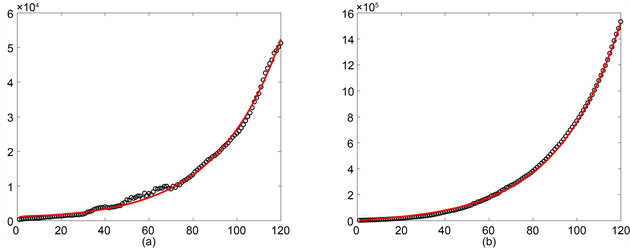Figure 3. Least squares were used to fit India’s case data from April 1, 2020, to July 27, 2020. (a) New cases; (b) Cumulative cases

4.2. 免疫比例x为常数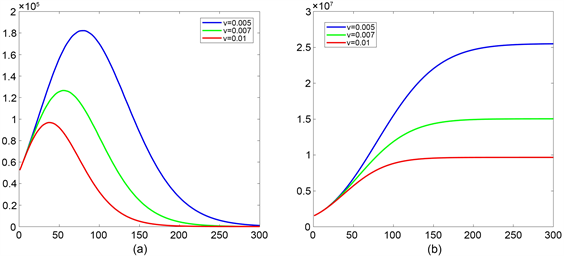Figure 4. With different v values, the patient’s trajectory over time. (a) New cases; (b) Cumulative cases

4.3. 疫苗博弈的免疫效果模拟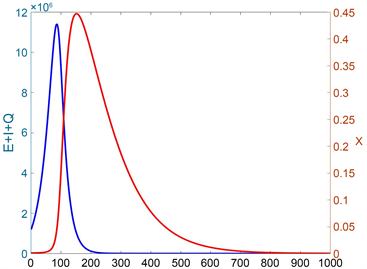Figure 5. Take $\omega =0.000001,k=0.01$, the curve that the patient and the vaccinator change over time after the vaccine game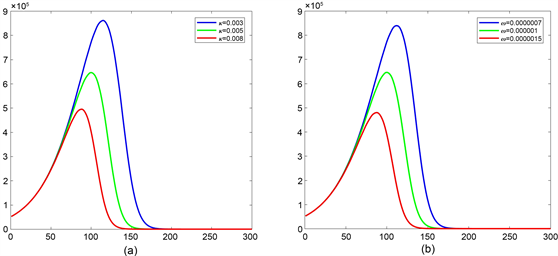Figure 6. Joining the game vaccine immunization from the end of July 2020. (a) In $\omega =0.000001$, new cases under different $\kappa$ values; (b) In $\kappa =0.0\text{05}$, new cases under different $\omega$ values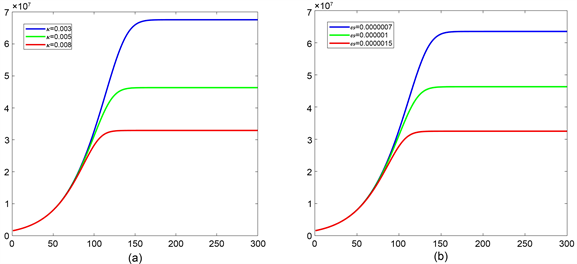Figure 7. Joining the game vaccine immunization from the end of July 2020. (a) In $\omega =0.000001$, cumulative case under different $\kappa$ values; (b) In $\kappa =0.0\text{05}$, cumulative case under different $\omega$ values

4.4. 基本再生数R0和有效再生数Rv, Rω的比较

${v}_{0}=\frac{v\left(1+\mu \right)}{\mu +v},{\omega }_{0}=\frac{\left(\delta +\mu \right)\left(\gamma +\mu \right)\left(m+\mu \right)}{\mu \omega N\left(\mu {R}_{0}+1\right)\left[\left(\gamma +\mu \right)\left(m+\mu \right)+\delta \left(\gamma +\mu \right)+\delta m\right]}.$Table 3. The expressions for R 0 , R v , R ω

5. 结论

 Gorbalenya, A.E., Baker, S.C., Baric, R.S., Groot, R.J.D. and Ziebuhr, J. (2020) The Species Severe Acute Respiratory Syndrome-Related Coronavirus: Classifying 2019-NCoV and Naming It SARS-CoV-2. Nature Microbiology, 5, 536-544.
https://doi.org/10.1038/s41564-020-0695-z

 谢识予. 经济博弈论[M]. 第2版. 上海: 复旦大学出版社, 2002: 23-40.

 Zhao, S., Stone, L., Gao, D., Musa, S.S. and Wang, M.H. (2020) Imitation Dynamics in the Mitigation of the Novel Coronavirus Disease (COVID-19) Outbreak in Wuhan, China from 2019 to 2020. Annals of Translational Medicine, 8, 448.
https://doi.org/10.21037/atm.2020.03.168

 APAKabir, A., and Tanimoto, J. (2020) Evolutionary Game Theory Modeling to Represent the Behavioral Dynamics of Economic Shutdowns and Shield Immunity in the COVID-19 Pandemic. Royal Society Open Science, 7, 1-8.
https://doi.org/10.1098/rsos.201095

 Wei J. Y., Wang L., Yang X. (2020) Game Analysis on the Evolution of COVID-19 Epidemic under the Prevention and Control Measures of the Government. PloS One, 15, e0240961.
https://doi.org/10.1371/journal.pone.0240961

 Bauch, C. T. (2005) Imitation Dynamics Predict Vaccinating Behaviour. Proceedings Biological Sciences, 272, 1669-1675.
https://doi.org/10.1098/rspb.2005.3153

 Diekmann, O., Heesterbeek, J.A.P. and Metz, J.A.J. (1990) On the Definition and the Computation of the Basic Reproduction Ratio R0 in Models for Infectious Diseases in Heterogeneous Populations. Journal of Mathematical Biology, 28, 365-382.
https://doi.org/10.1007/BF00178324

 Dreessche, P. and Watmough, J. (2002) Reproduction Numbers and Sub-Threshold Endemic Equilibria for Compartmental Models of Disease Transmission. Mathematical Biosciences, 180, 29-48.
https://doi.org/10.1016/S0025-5564(02)00108-6

 Lasalle, J.P. (1976) The Stability of Dynamical Systems. Society for Industrial and Applied Mathematics, Philadelphia.

 Li, M.Y. and Shuai, Z. (2010) Global-Stability Problem for Coupled Systems of Differential Equations on Networks. Journal of Differential Equations, 248, 1-20.
https://doi.org/10.1016/j.jde.2009.09.003

Top